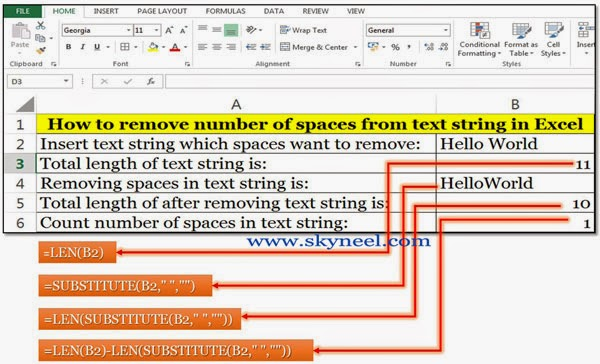# How to count total White Spaces in Text String MS Excel

If you want to count white spaces in a text string then you must read this article-How to count total White Spaces in Text String.

Hello friends all we know very well about the importance of MS Excel. In daily official jobs we must have to use MS Excel for preparing different kind of MIS reports on which we have to apply different commands and functions to get required results. Today we have to discuss MS Excel-how to count total white spaces in text string.

Today we have to discuss about the importance of Count function in MS Excel. With the help of count function you will be able to easily count white spaces in any text string. Lot of time we, need to count number of whites spaces within given text string but main problem is that how to count total number of spaces in a text string in active cell in Microsoft Excel. You can try this facility in excel to overcome your problem regarding counting number of spaces in a string using formula in excel sheet. It will make improve your efficiency and experience, it is much easier and enjoyable.

## How to count total White Spaces in Text String

If you have number of spaces in any text string and now you want to count all those spaces, then you must read this article MS Excel-how to count total white spaces in text string.

Step 1: Prepare the following worksheet in MS-Excel given below.

Step 2: Apply the following formulas in cell B3, B4, B5 & B6.Step 3: How to Know Total Length of Text String: You can use LEN function, it is an important text function which is used to return number of characters within a text string. This function also count all spaces given within text string.

The syntax of this function is: =LEN (text).

For example, if you type any text in cell B2 and want to calculate total length of this cell, you type following formula in another cell like in cell B3.

=LEN(B2)

Step 4: How to Removing Number of Spaces from Text String: Substitutes function, it is an important text function which is used to remove any old text with the new text within a given text string. For example, if you want to remove number of spaces from text string of cell B2 then you have to type following given formula in any other cell like in cell B4.

=SUBSTITUTE(B2,” “,””)

Step 5: How To Know Total Length of after Removing Spaces in Text String: If you want to know total length and also remove all number of spaces from the text string then you have to merge both LEN and SUBSTITUTE function like given below in cell B5.

=LEN(SUBSTITUTE(B2,” “,””))

Step 6: How to Count number of Spaces in Text String: If you want to count number of spaces within a given text string you have to merge LEN and SUBSTITUTE functions both and prepare following function like given below in cell B6.

=LEN(B2)-LEN(SUBSTITUTE(B2,” “,””))

I hope how to count total white spaces in text string article will be helpful for all the users. Kindly share your knowledge with your family members, colleague and others. If you have any suggestion regarding this article then write us in the comment box. Thanks to all of you.